# Retrieve time period where a certain value matchesHi, Anaplaners,

I am struggling with how I can retrieve Time Period where num1 matched to num2 (please see the attached file for the detail)

(cell colors in the file do not intend conditional formatting)

Tagged:

•Hi Gregory,

I Assumed your model contains 2 years data, So total quarterly no will be 8(2*4) records.

I created a list as shown below,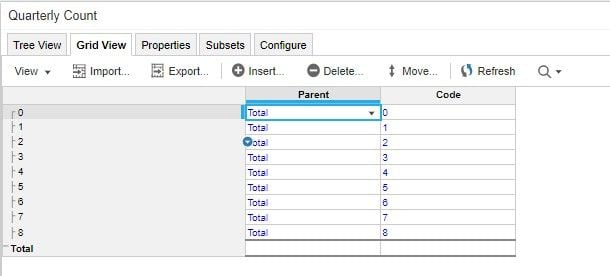Then Create a module with 3 line item and Quarterly Count list to find the quarter, as shown belowFormulas in Line item,

A = PERIOD(START()) + VALUE(NAME(ITEM(Quarterly Count)))

B = Retrieve time period.'Num 2' = Retrieve time period.'Num 1'[LOOKUP: A]

C = IF B THEN A ELSE BLANK

In Final Module, pick the data from the "C" line item.Grid View,Try this solution.

~Vignesh

•Hi Gregory,

Im not sure how you get Num 2 calculation, but I propose to you my solution below:

1) Module view screenshot with Result: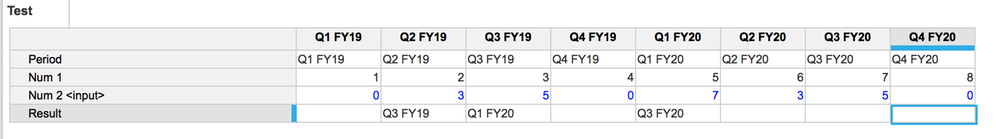2) Blue print of the module: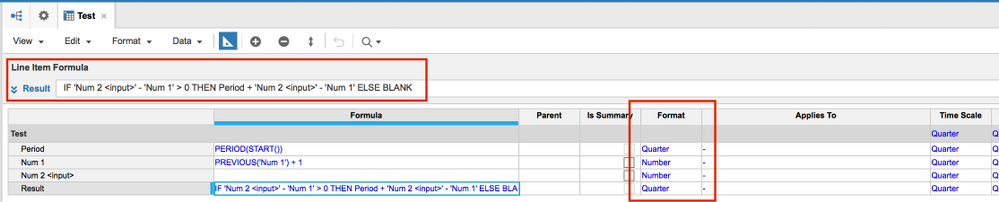Description:

- First I created line item which will indetify current period for each quarter. lineitem format is Time period - Quarter

- Num 1 populated with formula to generate 1,2,3,4...

- Result line item referenced to Period and adds diffrence between Num 1 and Num 2

IF 'Num 2 <input>' - 'Num 1' > 0   //Herewe can also change to 'Num 2 <input>'   <> 0

THEN

Period + 'Num 2 <input>' - 'Num 1'  // There we adding amount of periods to current period

ELSE

BLANK  // If condition is false - we will leave time periods as blank

Regards, Nikolay

•Please remember we can do some very simple formulas with time

Assuming that the example is a real Anaplan timescale you can achieve the result with a very simple addition

QTR1 FY19 + 1 = QTR2 FY19 it's as simple as that

Jan 19 + 1 = Feb 19

FY19 + 1 = FY20

so: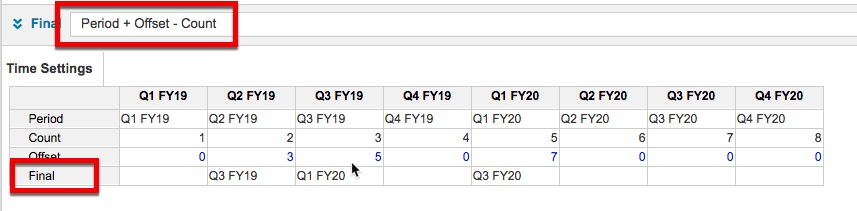The Period line item is formatted as Time Period:Quarter and the formula is ITEM(Time)

The Count line item formula is CUMULATE(1).

For long detailed timescales you should use Count +PREVIOUS(Count)

Also if the Offset line item is driven from more dimensions, the Period and Count line items should be stored in you Time Settings module and referenced in the target module

David

•Hi Gregory,

I assumed the data in NUM 1 and NUM2 can be any numbers, so I tried it in a different way,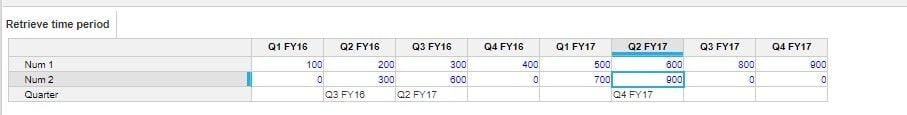if the NUM1 and NUM 2 are random numbers, then try this solution.

~Vignesh

•Thank you All for your replies.

Apologies for the confusion that Ive caused. Num1 is not sequential numbers, but randam numbers. (please see the new file)

•@gregoryTan No apology needed, but as with most problems, please could you explain the wider context of what you are trying to do and why; it does help drive the correct solution

David

••Thank you David. I will try to generalize my query when I start a topic next time.

•Apoligies for the delay, but I have a solution to the problem that is a bit simpler and will take up fewer model cells.

As background, due to the way the Anaplan calc engine defines blocks it is not possible to easily SUM from a module with time to one without. Hence we have to get a little creative with dummy timescales and move the data around

1. Firstly create a list of "numbers".  This should cover the maximum number of periods the user can enter.  I have also taken the assumption that the users woukld enter a number as a number rather than a drop down list.  So we need to convert the entered number into the list number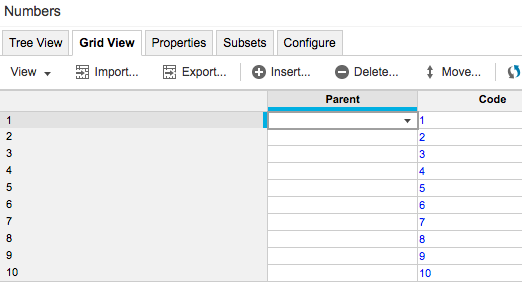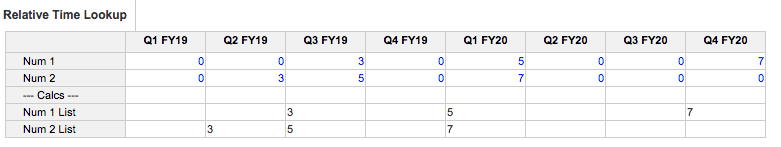The formulas for Num 1 List and Num 2 List are:

FINDITEM(Numbers, TEXT('Num 1')) and FINDITEM(Numbers, TEXT('Num 2'))

2. Create a dummy timescale to match the real timescale (you only need the detailed members) and I would always suggest using the same display format as the real timescale (it makes the next piece easier)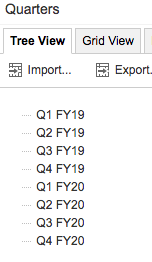3. We now need to map the number list from 1. into a module by the dummy Quarter list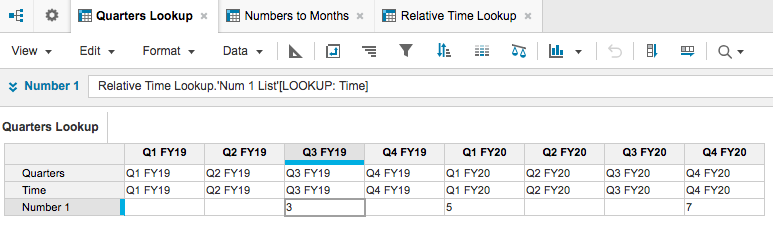The Blueprint is: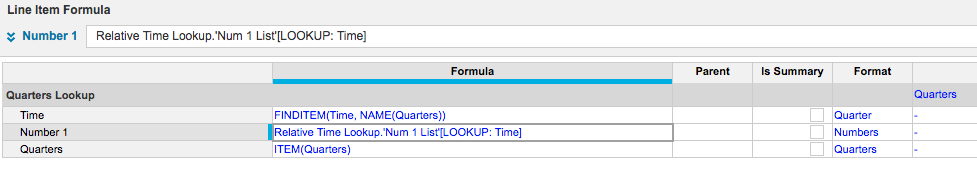4. We now create a module by our numbers list and map in the relavant quarter (from the dummry Quarters list)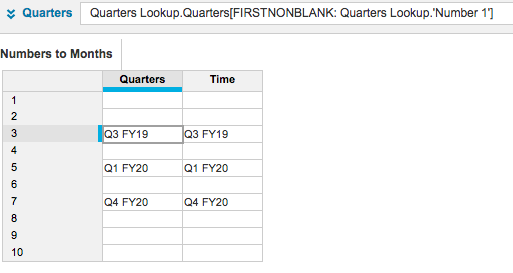The formula for the Time Line item is FINDITEM(Time, NAME(Quarters)).  This is the last peice of the puzzle as we now have the mapping between numbers (list) and time periods (real timescale)

5. We can now use the module from above to map in the num 2 choice: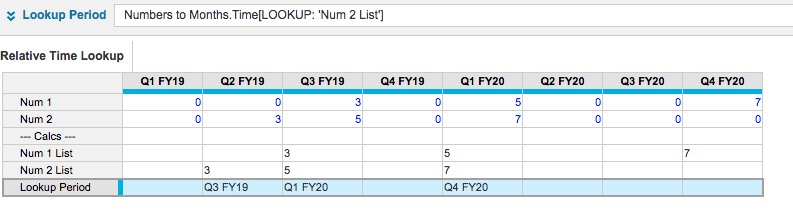Happy modelling!

David